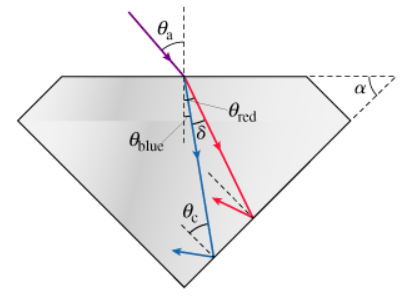# Problem: A beam of white light is incident on the surface of a diamond at an angle θa. (Figure 1) Since the index of refraction depends on the light's wavelength, the different colors that comprise white light will spread out as they pass through the diamond. The indices of refraction in diamond are nred = 2.410 for red light and nblue = 2.450 for blue light. The surrounding air has nair = 1.000. Note that the angles in the figure are not to scale.Derive a formula for δ, the angle between the red and blue refracted rays in the diamond. Express the angle in terms of nred, nblue, and θa. Use nair = 1.

###### FREE Expert Solution

Snell's law:

θ1 is the angle of incidence and θ2 is the angle of refraction.

sinθ2 = (η12)sinθ1

θ2 = sin−1 ((η12)sinθ1)

96% (12 ratings)###### Problem Details

A beam of white light is incident on the surface of a diamond at an angle θa. (Figure 1) Since the index of refraction depends on the light's wavelength, the different colors that comprise white light will spread out as they pass through the diamond. The indices of refraction in diamond are nred = 2.410 for red light and nblue = 2.450 for blue light. The surrounding air has nair = 1.000. Note that the angles in the figure are not to scale.Derive a formula for δ, the angle between the red and blue refracted rays in the diamond. Express the angle in terms of nred, nblue, and θa. Use nair = 1.